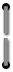# Appendix D

### Operating Limits

##### Accuracy
Accuracy of the HP-80 varies according to the operation being performed.Arithmetic operations (+, –, ×, ÷) are accurate to 10 digits ± 1 count; that is, the value of the 10th digit could be 1 less or I more than the value displayed.Special operations solved through an iterative method (i(YTM) andi)   use numerical analysis techniques formerly requiring computers or large-scale programmable calculators. These operations are accurate to 5 digits.The yx function (which is used in the top row financial operations) produces an answer corresponding to some x within ± 7 counts in the tenth least significant digit of the input x, and some y within ± 4 counts in the tenth least significant digit of the input y.
##### Execution Time
Execution time for iterative solutions varies according to the numbers you enter. For example, in certain interest rate calculations the solution time is related to the ratio PV/(PMT × n) or FV/(PMT × n). Extreme cases outside those normally encountered may require minutes.
##### Range
Financial calculations using the top row of keys are not permitted for interest rate values very close or equal to 0; specifically, rates between i= –0.0000001 % and i= + 0.0000001 % are not handled.
##### Range Errors
Any computation or data entry resulting in a magnitude greater than or equal to 1099, or less than 10–99, triggers an error signal indicated by a blinking display.
 Bond yield calculations may result in slightly reduced accuracy if the problem is an unusual case such as calculating a 50% yield-to-maturity for a 2% bond. For example, the yield-to-maturity on a 2% bond, maturing in 3 years, 3 months and selling at 26.481133 is 50.00%. The answer displayed is 49.99%. The formula for determining error magnitude is, percent calculation error for bond yield ≤ 2 × yield (actual) × coupon × 10–4. The operating limits for the bond yield algorithm are defined such that the price of the bond must be above 20 and below 5,000, and coupon must be greater than 1/8% and less than the price of the bond (expressed as a percent of par).67